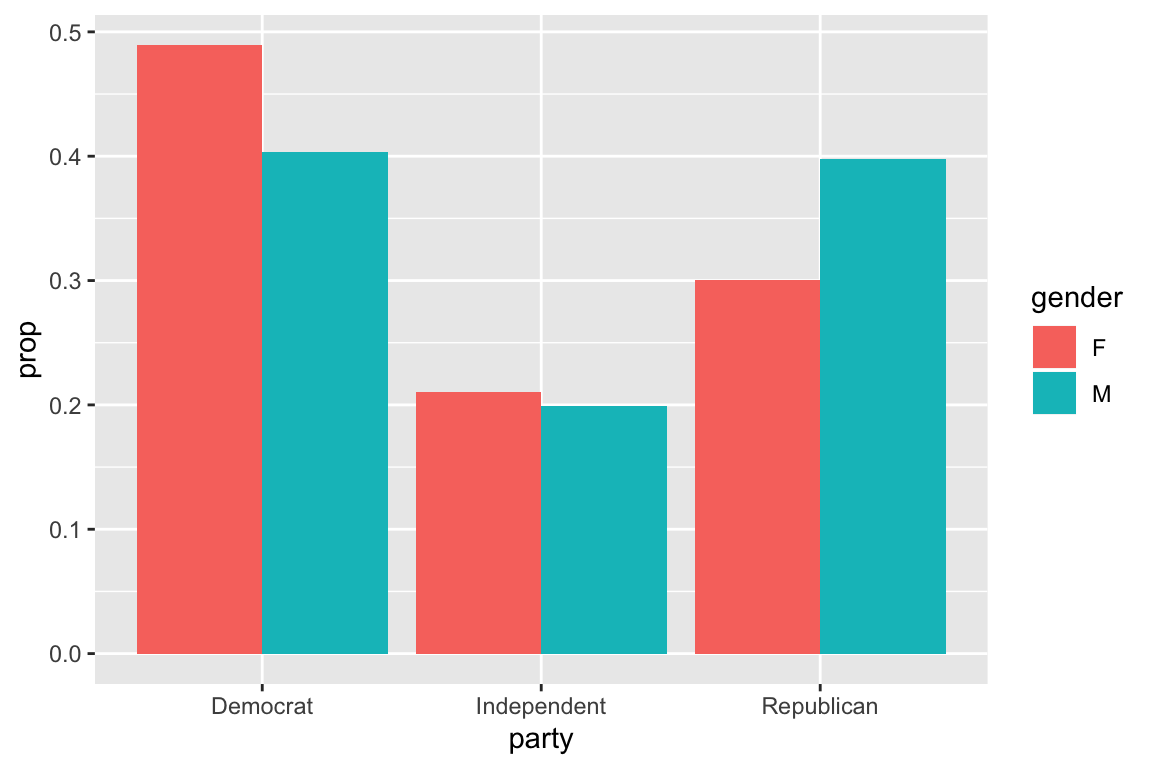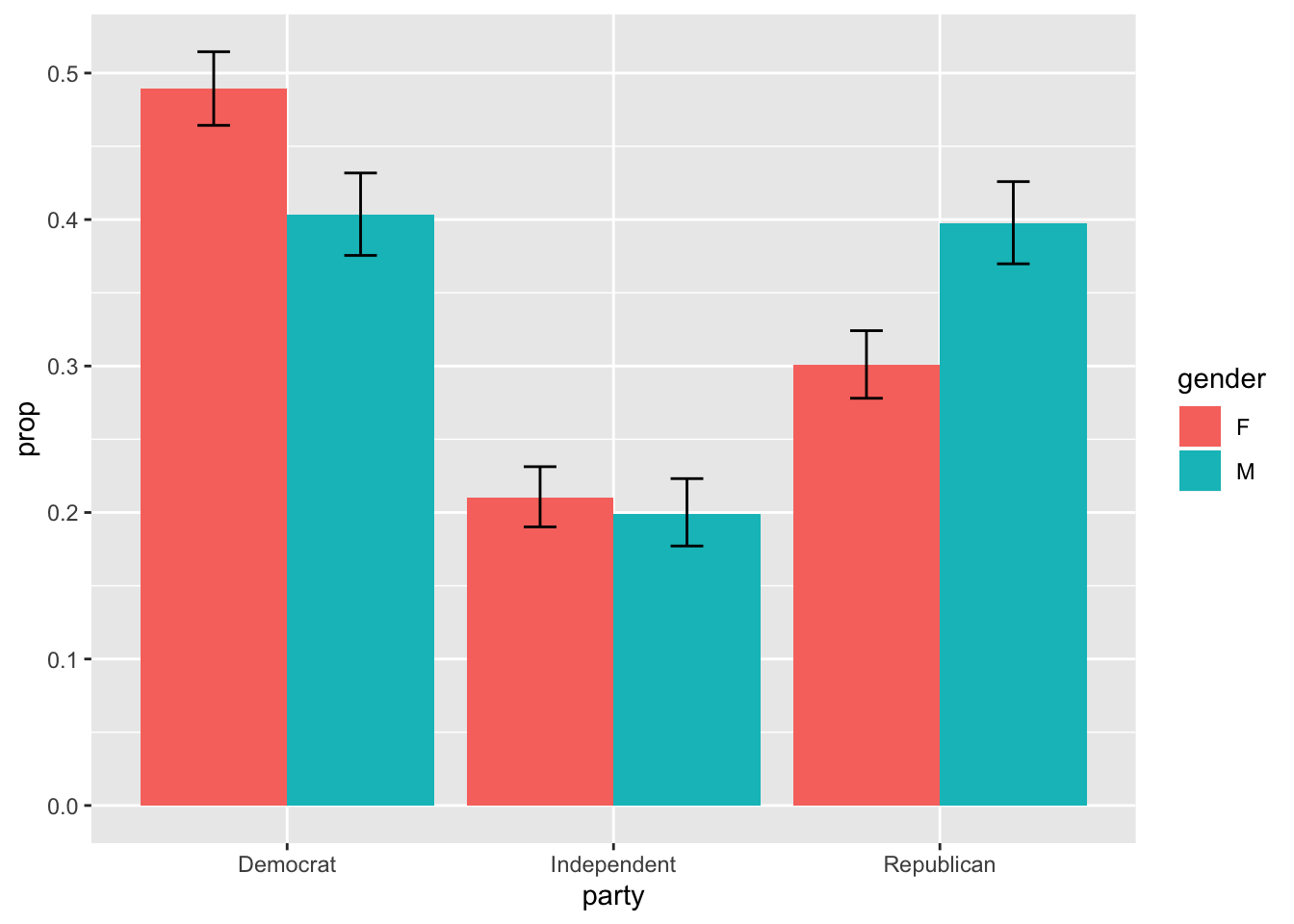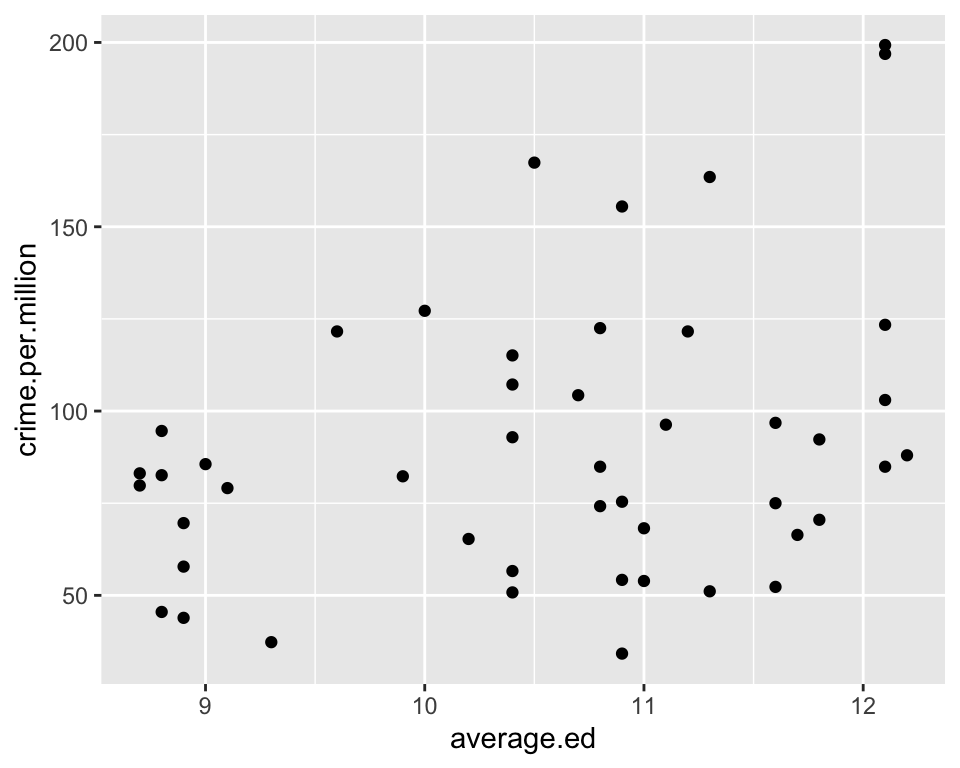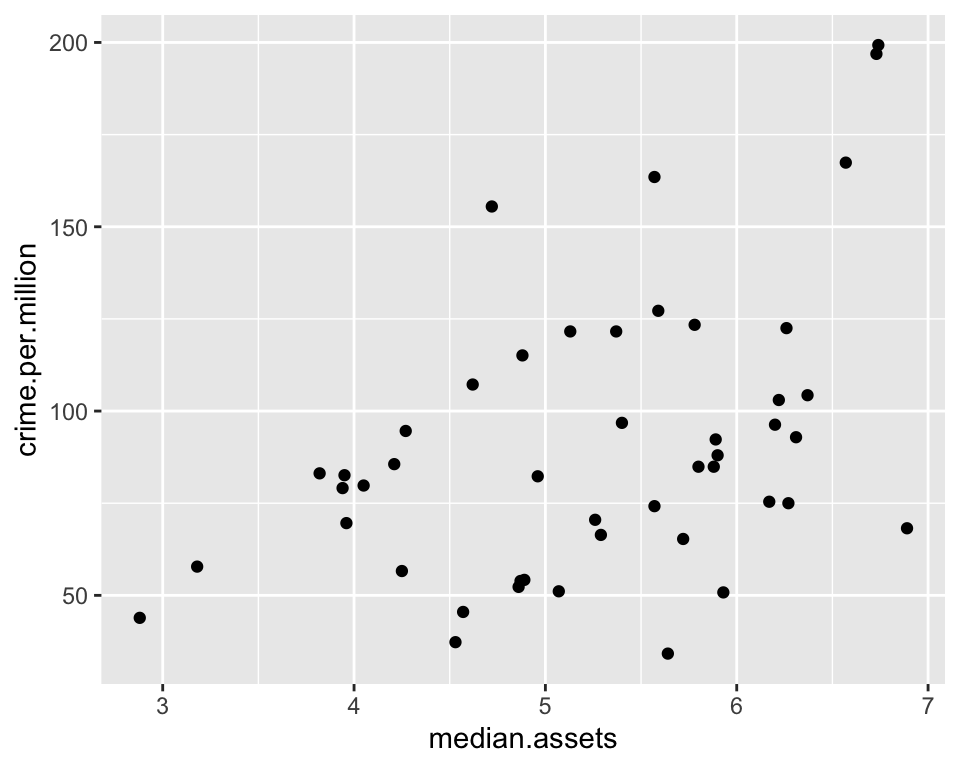### Agenda

• 2x2 tables, j x k tables

• ANOVA

• Linear regression
• Fitting linear regression models in R
• Diagnostic plots
• Interpreting regression coefficients
• Testing significance of factor variables

library(tidyverse)
## ── Attaching packages ─────────────────────────────────────────────────── tidyverse 1.2.1 ──
## ✔ ggplot2 3.2.1     ✔ purrr   0.3.3
## ✔ tibble  2.1.3     ✔ dplyr   0.8.3
## ✔ tidyr   1.0.0     ✔ stringr 1.4.0
## ✔ readr   1.3.1     ✔ forcats 0.4.0
## ── Conflicts ────────────────────────────────────────────────────── tidyverse_conflicts() ──
## ✖ dplyr::lag()    masks stats::lag()
library(knitr)

We’ll begin by doing all the same data processing as in previous lectures

# Load data from MASS into a tibble
birthwt <- as_tibble(MASS::birthwt)

# Rename variables
birthwt <- birthwt %>%
rename(birthwt.below.2500 = low,
mother.age = age,
mother.weight = lwt,
mother.smokes = smoke,
previous.prem.labor = ptl,
hypertension = ht,
uterine.irr = ui,
physician.visits = ftv,
birthwt.grams = bwt)

# Change factor level names
birthwt <- birthwt %>%
mutate(race = recode_factor(race, 1 = "white", 2 = "black", 3 = "other")) %>%
mutate_at(c("mother.smokes", "hypertension", "uterine.irr", "birthwt.below.2500"),
~ recode_factor(.x, 0 = "no", 1 = "yes"))

### Tests for 2x2 tables

Here’s an example of a 2 x 2 table that we might want to run a test on. This one looks at low birthweight broken down by mother’s smoking status. You can think of it as another approach to the t-test problem, this time looking at indicators of low birth weight instead of the actual weights.

First, let’s build our table using the table() function (we did this back in Lecture 5)

weight.smoke.tbl <- with(birthwt, table(birthwt.below.2500, mother.smokes))
weight.smoke.tbl
##                   mother.smokes
## birthwt.below.2500 no yes
##                no  86  44
##                yes 29  30

When we have this type of tabular data we are interested in testing: Is there an association between the row variables and the column variables? i.e., If I tell you whether the mother smoked during pregnancy, does that inform you as to whether the baby is more or less likely to be born with low birthweight? Conversely, if I tell you the baby was both with low birthweight, does that inform you as to the mother’s likely smoking status during pregnancy?

To test for significance, we just need to pass our 2 x 2 table into the appropriate function. Here’s the result of using fisher’s exact test by calling fisher.test

birthwt.fisher.test <- fisher.test(weight.smoke.tbl)
birthwt.fisher.test
##
##  Fisher's Exact Test for Count Data
##
## data:  weight.smoke.tbl
## p-value = 0.03618
## alternative hypothesis: true odds ratio is not equal to 1
## 95 percent confidence interval:
##  1.028780 3.964904
## sample estimates:
## odds ratio
##   2.014137
attributes(birthwt.fisher.test)
## $names ##  "p.value" "conf.int" "estimate" "null.value" "alternative" ##  "method" "data.name" ## ##$class
##  "htest"

The fisher test is a formal test of independence. The null is that the row variable and column variable are statistically independent. Instead of testing differences in means, the natural thing to test in 2x2 tables is whether the odds ratio is equal to 1. Let $$p_{s} = P(\text{low birthweight} \mid \text{smoke})$$ (the probability of low birthweight among smoking mothers) and let $$p_{n} = P(\text{low birthweight} \mid \text{doesn't smoke})$$ (the probability of low birthweight among nonsmoking mothers). The odds of low weight among smoking mothers is defined as:

$\mathrm{odds}_s = \frac{p_s}{1 - p_s}$ The odd ratio (OR) summarizes the association between smoking and low birthweight. It is defined as the odds of low birthweight under smoking divided by the odds under not smoking.

$\mathrm{OR} = \frac{p_s}{1 - p_s} \Big/ \frac{p_n}{1 - p_n}$

Interpretation: The odds of low birth weight are 2.01 times greater when the mother smokes than when the mother does not smoke.

You can also use the chi-squared test via the chisq.test function. This is the test that you may be more familiar with from your statistics class.

chisq.test(weight.smoke.tbl)
##
##  Pearson's Chi-squared test with Yates' continuity correction
##
## data:  weight.smoke.tbl
## X-squared = 4.2359, df = 1, p-value = 0.03958

You get essentially the same answer by running the chi-squared test, but the output isn’t as useful. In particular, you’re not getting an estimate or confidence interval for the odds ratio. This is why I prefer fisher.exact() for testing 2 x 2 tables.

#### Tests for j x k tables

Here’s a small data set on party affiliation broken down by gender.

# Manually enter the data
politics <- as.table(rbind(c(762, 327, 468), c(484, 239, 477)))
dimnames(politics) <- list(gender = c("F", "M"),
party = c("Democrat","Independent", "Republican"))

politics # display the data
##       party
## gender Democrat Independent Republican
##      F      762         327        468
##      M      484         239        477

We may be interested in asking whether men and women have different party affiliations.

The answer will be easier to guess at if we convert the rows to show proportions instead of counts. Here’s one way of doing this.

politics.prop <- prop.table(politics, 1)
politics.prop
##       party
## gender  Democrat Independent Republican
##      F 0.4894027   0.2100193  0.3005780
##      M 0.4033333   0.1991667  0.3975000

By looking at the table we see that Female are more likely to be Democrats and less likely to be Republicans.

We still want to know if this difference is significant. To assess this we can use the chi-squared test (on the counts table, not the proportions table!).

chisq.test(politics)
##
##  Pearson's Chi-squared test
##
## data:  politics
## X-squared = 30.07, df = 2, p-value = 2.954e-07

There isn’t really a good one-number summary for general $$j$$ x $$k$$ tables the way there is for 2 x 2 tables. One thing that we may want to do at this stage is to ignore the Independent category and just look at the 2 x 2 table showing the counts for the Democrat and Republican categories.

politics.dem.rep <- politics[,c(1,3)]
politics.dem.rep
##       party
## gender Democrat Republican
##      F      762        468
##      M      484        477
# Run Fisher's exact test
fisher.test(politics.dem.rep)
##
##  Fisher's Exact Test for Count Data
##
## data:  politics.dem.rep
## p-value = 6.806e-08
## alternative hypothesis: true odds ratio is not equal to 1
## 95 percent confidence interval:
##  1.347341 1.910944
## sample estimates:
## odds ratio
##   1.604345

We see that women have significantly higher odds of being Democrat compared to men.

### Plotting the table values with confidence

It may be useful to represent the data graphically. Here’s one way of doing so with the ggplot2 package. Note that we plot the proportions not the counts.

1. Convert the table into something ggplot2 can process

politics.prop
##       party
## gender  Democrat Independent Republican
##      F 0.4894027   0.2100193  0.3005780
##      M 0.4033333   0.1991667  0.3975000
politics.df <- as_tibble(politics.prop)
politics.df
## # A tibble: 6 x 3
##   gender party           n
##   <chr>  <chr>       <dbl>
## 1 F      Democrat    0.489
## 2 M      Democrat    0.403
## 3 F      Independent 0.210
## 4 M      Independent 0.199
## 5 F      Republican  0.301
## 6 M      Republican  0.398
# Change n to a less confusing name
politics.df <- politics.df %>%
rename(prop = n)

2. Create a ggplot2 object, and plot with geom_barplot()

ggplot(politics.df, aes(x=party, y=prop, fill=gender)) +
geom_bar(position="dodge", stat="identity")This figure is a nice alternative to displaying a table. One thing we might want to add is a way of gauging the statistical significance of the differences in height. We’ll do so by adding error bars.

#### Adding error bars to bar plots

Remember, ggplot wants everything you plot to be sitting nicely in a data frame. Here’s some code that will calculate the relevant values and do the plotting.

1. Get the data into a form that’s easy to work with.

# Form into a long data frame
politics.df.count <- as_tibble(politics)
# print
politics.df.count
## # A tibble: 6 x 3
##   gender party           n
##   <chr>  <chr>       <dbl>
## 1 F      Democrat      762
## 2 M      Democrat      484
## 3 F      Independent   327
## 4 M      Independent   239
## 5 F      Republican    468
## 6 M      Republican    477
# Add a column of marginal counts
politics.df.count <- politics.df.count %>%
group_by(gender) %>%
mutate(totals = sum(n))

# print
politics.df.count
## # A tibble: 6 x 4
## # Groups:   gender 
##   gender party           n totals
##   <chr>  <chr>       <dbl>  <dbl>
## 1 F      Democrat      762   1557
## 2 M      Democrat      484   1200
## 3 F      Independent   327   1557
## 4 M      Independent   239   1200
## 5 F      Republican    468   1557
## 6 M      Republican    477   1200

2. Calculate confidence intervals.

To calculate confidence intervals for the proportions, we can use prop.test or binom.test. Essentially you call these functions the numerator and denominator for the proportion.

We’ll do this with a ddply call. First, let’s look at an example of how the prop.test works.

# Suppose that we had 80 coin flips, 27 of which came up heads.
# How can we get a 95% CI for the true probability that this coin comes up H?
coin.test <- prop.test(27, 80)
coin.test
##
##  1-sample proportions test with continuity correction
##
## data:  27 out of 80, null probability 0.5
## X-squared = 7.8125, df = 1, p-value = 0.005189
## alternative hypothesis: true p is not equal to 0.5
## 95 percent confidence interval:
##  0.2379394 0.4528266
## sample estimates:
##      p
## 0.3375
coin.test$conf.int ##  0.2379394 0.4528266 ## attr(,"conf.level") ##  0.95 politics.toplot <- politics.df.count %>% group_by(gender, party) %>% summarize(prop = n / totals, lower = prop.test(n, totals)$conf.int,
upper = prop.test(n, totals)$conf.int) politics.toplot ## # A tibble: 6 x 5 ## # Groups: gender  ## gender party prop lower upper ## <chr> <chr> <dbl> <dbl> <dbl> ## 1 F Democrat 0.489 0.464 0.515 ## 2 F Independent 0.210 0.190 0.231 ## 3 F Republican 0.301 0.278 0.324 ## 4 M Democrat 0.403 0.376 0.432 ## 5 M Independent 0.199 0.177 0.223 ## 6 M Republican 0.398 0.370 0.426 3. Combine the confidence intervals into the data frame 4. Use ggplot(), geom_bar() and geom_errorbar() to construct the plots ggplot(politics.toplot, aes(x=party, y=prop, fill=gender)) + geom_bar(position="dodge", stat="identity") + geom_errorbar(aes(ymin=lower, ymax=upper), width=.2, # Width of the error bars position=position_dodge(0.9))### ANOVA You can think of ANOVA (analysis of variance) as a more general version of the t-test, or a special case of linear regression in which all covariates are factors. Let’s go back to our favourite birthwt data set from the MASS library. #### One-way ANOVA example Question: Is there a significant association between race and birthweight? Here’s a table showing the mean and standard error of birthweight by race. birthwt %>% group_by(race) %>% summarize(mean.bwt = round(mean(birthwt.grams), 0), se.bwt = round(sd(birthwt.grams) / sqrt(n()), 0)) ## # A tibble: 3 x 3 ## race mean.bwt se.bwt ## <fct> <dbl> <dbl> ## 1 white 3103 74 ## 2 black 2720 125 ## 3 other 2805 88 It looks like there’s some association, but we don’t yet know if it’s statistically significant. Note that if we had just two racial categories in our data, we could run a t-test. Since we have more than 2, we need to run a 1-way analysis of variance (ANOVA). Terminology: a $$k$$-way ANOVA is used to assess whether the mean of an outcome variable is constant across all combinations of $$k$$ factors. The most common examples are 1-way ANOVA (looking at a single factor), and 2-way ANOVA (looking at two factors). We’ll use the aov() function. For convenience, aov() allows you to specify a formula. summary(aov(birthwt.grams ~ race, data = birthwt)) ## Df Sum Sq Mean Sq F value Pr(>F) ## race 2 5015725 2507863 4.913 0.00834 ** ## Residuals 186 94953931 510505 ## --- ## Signif. codes: 0 '***' 0.001 '**' 0.01 '*' 0.05 '.' 0.1 ' ' 1 The p-value is significant at the 0.05 level, so the data suggests that there is an association between birthweight and race. In other words, average birthweight varies across the three racial groups considered in the data. ### Linear regression Linear regression is just a more general form of ANOVA. It allows you to model effects of continuous variables. linear regression is used to model linear relationship between an outcome variable, $$y$$, and a set of covariates or predictor variables $$x_1, x_2, \ldots, x_p$$. For our first example we’ll look at a small data set in which we’re interested in predicting the crime rate per million population based on socio-economic and demographic information at the state level. Let’s first import the data set and see what we’re working with. # Import data set crime <- read_delim("http://www.andrew.cmu.edu/user/achoulde/94842/data/crime_simple.txt", delim = "\t") ## Parsed with column specification: ## cols( ## R = col_double(), ## Age = col_double(), ## S = col_double(), ## Ed = col_double(), ## Ex0 = col_double(), ## Ex1 = col_double(), ## LF = col_double(), ## M = col_double(), ## N = col_double(), ## NW = col_double(), ## U1 = col_double(), ## U2 = col_double(), ## W = col_double(), ## X = col_double() ## ) The variable names that this data set comes with are very confusing, and even misleading. R: Crime rate: # of offenses reported to police per million population Age: The number of males of age 14-24 per 1000 population S: Indicator variable for Southern states (0 = No, 1 = Yes) Ed: Mean # of years of schooling x 10 for persons of age 25 or older Ex0: 1960 per capita expenditure on police by state and local government Ex1: 1959 per capita expenditure on police by state and local government LF: Labor force participation rate per 1000 civilian urban males age 14-24 M: The number of males per 1000 females N: State population size in hundred thousands NW: The number of non-whites per 1000 population U1: Unemployment rate of urban males per 1000 of age 14-24 U2: Unemployment rate of urban males per 1000 of age 35-39 W: Median value of transferable goods and assets or family income in tens of$

X: The number of families per 1000 earning below 1/2 the median income

We really need to give these variables better names

# Assign more meaningful variable names, also
# Convert is.south to a factor
# Divide average.ed by 10 so that the variable is actually average education
# Convert median assets to 1000's of dollars instead of 10's
crime <- crime %>%
rename(crime.per.million = R,
young.males = Age,
is.south = S,
average.ed = Ed,
exp.per.cap.1960 = Ex0,
exp.per.cap.1959 = Ex1,
labour.part = LF,
male.per.fem = M,
population = N,
nonwhite = NW,
unemp.youth = U1,
median.assets = W,
num.low.salary = X) %>%
mutate(is.south = as.factor(is.south),
average.ed = average.ed / 10,
median.assets = median.assets / 100)
# print summary of the data
summary(crime)
##  crime.per.million  young.males    is.south   average.ed
##  Min.   : 34.20    Min.   :119.0   0:31     Min.   : 8.70
##  1st Qu.: 65.85    1st Qu.:130.0   1:16     1st Qu.: 9.75
##  Median : 83.10    Median :136.0            Median :10.80
##  Mean   : 90.51    Mean   :138.6            Mean   :10.56
##  3rd Qu.:105.75    3rd Qu.:146.0            3rd Qu.:11.45
##  Max.   :199.30    Max.   :177.0            Max.   :12.20
##  exp.per.cap.1960 exp.per.cap.1959  labour.part     male.per.fem
##  Min.   : 45.0    Min.   : 41.00   Min.   :480.0   Min.   : 934.0
##  1st Qu.: 62.5    1st Qu.: 58.50   1st Qu.:530.5   1st Qu.: 964.5
##  Median : 78.0    Median : 73.00   Median :560.0   Median : 977.0
##  Mean   : 85.0    Mean   : 80.23   Mean   :561.2   Mean   : 983.0
##  3rd Qu.:104.5    3rd Qu.: 97.00   3rd Qu.:593.0   3rd Qu.: 992.0
##  Max.   :166.0    Max.   :157.00   Max.   :641.0   Max.   :1071.0
##  Min.   :  3.00   Min.   :  2.0   Min.   : 70.00   Min.   :20.00
##  1st Qu.: 10.00   1st Qu.: 24.0   1st Qu.: 80.50   1st Qu.:27.50
##  Median : 25.00   Median : 76.0   Median : 92.00   Median :34.00
##  Mean   : 36.62   Mean   :101.1   Mean   : 95.47   Mean   :33.98
##  3rd Qu.: 41.50   3rd Qu.:132.5   3rd Qu.:104.00   3rd Qu.:38.50
##  Max.   :168.00   Max.   :423.0   Max.   :142.00   Max.   :58.00
##  median.assets   num.low.salary
##  Min.   :2.880   Min.   :126.0
##  1st Qu.:4.595   1st Qu.:165.5
##  Median :5.370   Median :176.0
##  Mean   :5.254   Mean   :194.0
##  3rd Qu.:5.915   3rd Qu.:227.5
##  Max.   :6.890   Max.   :276.0

#### First step: some plotting and summary statistics

You can start by feeding everything into a regression, but it’s often a better idea to construct some simple plots (e.g., scatterplots and boxplots) and summary statistics to get some sense of how the data behaves.

# Scatter plot of outcome (crime.per.million) against average.ed
qplot(average.ed, crime.per.million, data = crime)# correlation between education and crime
cor(crime$average.ed, crime$crime.per.million)
##  0.3228349

This seems to suggest that higher levels of average education are associated with higher crime rates. Can you come up with an explanation for this phenomenon?

# Scatter plot of outcome (crime.per.million) against median.assets
qplot(median.assets, crime.per.million, data = crime)# correlation between education and crime
cor(crime$median.assets, crime$crime.per.million)
##  0.4413199

There also appears to be a positive association between median assets and crime rates.